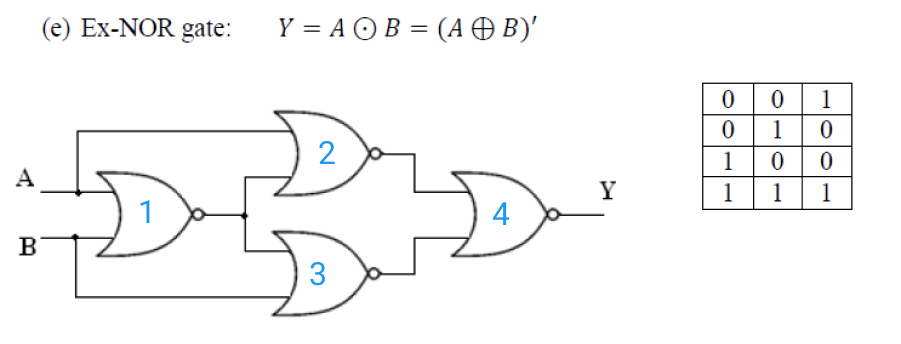# Implementation of EX-NOR Gate using NOR gate

<Back

## Aim

To study and verify the Implementation of EX-NOR Gate using NOR gate.

## Learning Objectives

1. To understand the behavior and demonstrate the Implementation of EX-NOR Gate using NOR gate.
2. To apply knowledge of the fundamental gates to create truth tables.
3. To develop digital circuit building and troubleshooting skills.
4. To understand key elements of TTL logic specification or datasheets.

## IC Used

For Implementation of EX-NOR Gate using NOR gate:
IC NumberIC Name

## Procedure

1. Place the IC on IC Trainer Kit.
2. Connect VCC and ground to respective pins of IC Trainer Kit.
3. Implement the circuit as shown in the circuit diagram.
4. Connect the inputs to the input switches provided in the IC Trainer Kit.
5. Connect the outputs to the switches of O/P LEDs
6. Apply various combinations of inputs according to the truth table and observe the condition of LEDs.
7. Note down the corresponding output readings for various combinations of inputs.
8. Power Off Trainer Kit, disconnect all the wire connections and remove IC's from IC-Base.

## Theory

Ex-NOR GATE - ​ The Exclusive-NOR Gate, also written as “Ex-NOR” or “XNOR”, function is achieved by combining standard gates together to form more complex gate functions.

The logic function implemented by a 2-input Ex-NOR gate is given as “when both A AND B are the SAME” will give an output at Q. In general, an Exclusive-NOR gate will give an output value of logic “1” ONLY when there are an EVEN number of 1’s on the inputs to the gate (the inverse of the Ex-OR gate) except when all its inputs are “LOW”.

Then an Ex-NOR function with more than two inputs is called an “even function” or modulo-2-sum (Mod-2-SUM), not an Ex-NOR.

Boolean Expression Y = (A ⊕ B)'NOR GATE - ​ It is a digital circuit that has two or more inputs and produces an output, which is the inversion of logical OR of all those inputs.

Logic NOR Gates are available using digital circuits to produce the desired logical function and is given a symbol whose shape is that of a standard OR gate with a circle, sometimes called an “inversion bubble” at its output to represent the NOT gate symbol with the logical operation of the NOR gate.

As with the OR function, the NOR function can also have any number of individual inputs and commercial available NOR Gate IC’s are available in standard 2, 3, or 4 input types. If additional inputs are required, then the standard NOR gates can be cascaded together to provide more inputs.

Boolean Expression Y = (​ A+B)​'

"If both A and B are NOT true, then Y is true"NOR gate operation is same as that of OR gate followed by an inverter. That’s why the NOR gate symbol is represented like that.

## Block Diagram## Precautions

1. Make the connections according to the IC pin diagram.
2. The connections should be tight on trainer kit.
3. The Vcc and ground should be applied carefully at the specified pin only.

## Conclusion

We have learned the Implementation of EX-NOR Gate using NOR gate.